Warning

# K-means Clustering

## Unsupervised Learning

The K-means algorithm is the first unsupervised method we encounter in the course. The previous supervised methods worked with a set of observations and labels $\mathcal{T} = \{(\mathbf{x}_1, y_1), \ldots, (\mathbf{x}_n, y_n)\}$. The unsupervised methods use unlabeled observations $\mathcal{T} = \{\mathbf{x}_1, \ldots, \mathbf{x}_n\}$. The goal is to infer properties of a probability distribution $p_X(\mathbf{x})$.

In low-dimensional problems ($|X| \leq 3$), we can estimate the probability density directly using variety of techniques (e.g. histograms, Parzen window). When we get prior information about the type of a particular probability distribution, we can directly estimate the distribution parameters.

Due to curse of dimensionality these methods fail in higher dimensions. When the dimensionality increases, the volume grows exponentially and the data becomes exponentially sparser. In such cases we use techniques that do not find the full underlying distribution $p_X(\mathbf{x})$, but instead describe the data in a more relaxed way.

The main groups of unsupervised methods are:

• dimensionality reduction methods
• clustering methods

The dimensionality reduction methods map data onto a lower-dimensional manifold while minimising the lost information. The clustering methods partition the observations into groups with small difference between the group samples.

The unsupervised methods have many applications including smart feature selection, data visualization, efficient data encoding, adaptive classification and image segmentation. Last but not least example is Google's PageRank algorithm.

## K-means

The K-means algorithm is a popular clustering approach. The goal is to find a clustering $\{\mathcal{T}_k\}^K_{k=1}$ of the observations that minimizes the within-cluster sum of squares (WCSS): $$\{\mathbf{c}_1, \ldots, \mathbf{c}_K; \mathcal{T}_1, \ldots, \mathcal{T}_K\} = \underset{\text{all }\mathbf{c}^{'}_k, \mathcal{T}^{'}_k} {\operatorname{arg\,min}} \sum_{k=1}^{K} \sum_{\mathbf{x} \in \mathcal{T}^{'}_k} \| \mathbf{x} - \mathbf{c}^{'}_k \|^2_2,$$ where $K$ is a user specified number of clusters, $\mathcal{T}_1,\ldots,\mathcal{T}_K$ are sets of observations belonging to clusters $1,\ldots,K$ and each cluster is represented by its prototype $\mathbf{c}_k$.

The K-means algorithm

Input: set of observations $\mathcal{T} = \{\mathbf{x}_1, \ldots, \mathbf{x}_n\}$, number of clusters $K$, maximum number of iterations.
Output: set of cluster prototypes $\{\mathbf{c}_1, \ldots, \mathbf{c}_K\}$.

Initialize $\{\mathbf{c}_1, \ldots, \mathbf{c}_K\}$ with random unique input observations $\mathbf{x}_i$.

Do:

1. Classify observations $\{\mathbf{x}_l\}^L_{l=1}$ to the cluster represented by the nearest prototype $\{\mathbf{c}_k\}^K_{k=1}$:
$$y_l = \arg\min_{k=1,\ldots,K}\|\mathbf{x}_l - \mathbf{c}_k\|^2_2 \qquad (\forall l = 1,\ldots,L),$$ $$\mathcal{T}_k = \{\mathbf{x} \in \mathcal{T}: \forall l, y_l = k\} \qquad (\forall k = 1,\ldots,K)$$

2. Update the prototypes $\mathbf{c}_k$ to the mean value of all vectors $\mathbf{x}_l$ belonging to $k$-th class:
$$\mathbf{c}_k = \frac{1}{|\mathcal{T}_k|}\sum_{\mathbf{x} \in \mathcal{T}_k} \mathbf{x}.$$

Until no change in clustering $\mathcal{T}_k$ or maximum number of iterations is exceeded.

### Optimality of the Solution

The solution that K-means reaches often depends on the initialisation of the means and there is no guarantee that it reaches the global optimum. As you can see in the two examples below, the solution is not unique and it can happen that the algorithm reaches a local minimum (as in the lower example), where reassigning any point to some other cluster would increase WCSS, but where a better solution does exist.

The chance of getting trapped in a local minimum can be reduced by performing more trials of the K-means clustering and choosing the result that minimizes WCSS.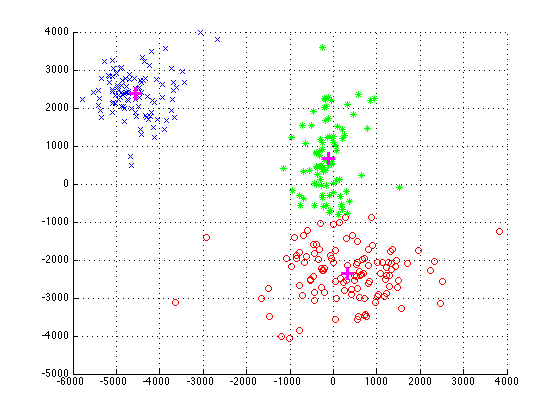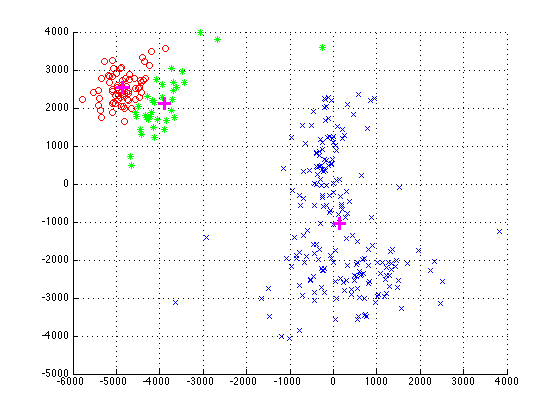### K-means++ initialization

K-Means++ is the K-means with clever initialization of cluster centers. The motivation is to make initializations which make K-means more likely to end up in better local minima (minima with lower WCSS).

K-Means++ uses the following randomized sampling strategy for constructing the initial cluster centers set $\mathcal{C}$:

1. Choose the first cluster centre $\mathbf{c}_1$ uniformly at random from $\mathcal{T}$. Set $\mathcal{C} = \{\mathbf{c}_1\}$.
2. For each data point $\mathbf{x}_l$, compute the distance $d_l$ to its nearest cluster in $\mathcal{C}$: $$d_l = \min_{\mathbf{c}\in \mathcal{C}} \|\mathbf{x}_l - \mathbf{c} \| \qquad (\forall l=1,2,..., L)$$
3. Select a point $\mathbf{x}_l$ from $\mathcal{T}$ with probability proportional to $d^2_l$. This involves constructing a distribution $p(l)$ from $d_l$ as $p(l) = \frac{d_l^2}{\sum_{l=1}^L d_l^2}$ and sampling from it to get the index $l$.
4. $\mathcal{C} \leftarrow \mathcal{C} \cup \mathbf{x}_l$.
5. Stop if $|\mathcal{C}| = K$, otherwise goto 2.

After this initialization, standard K-means algorithm is employed.

## Information for development in Python

To fulfil this assignment, you need to submit these files (all packed in a single .zip file) into the upload system:

• answers.txt - answers to the Assignment Questions
• assignment_10.m - a script for data initialization, calling of the implemented functions and plotting of their results (for your convenience, will not be checked)
• k_means.m - a function which implements the K-means algorithm
• k_means_multiple_trials.m - a function which performs several trials of the K-means clustering algorithm in order to avoid local minima
• k_meanspp.m, random_sample.m - functions for k-means++ initialization
• quantize_colors.m - a function which implements color quantization based on K-means
• random_initialization.png, kmeanspp_initialization.png, geeks_quantized.png - images specified in the tasks

## Information for development in Python

Experimental. Use at your own risk.

Standard is to use Matlab. You may skip this info box if you follow the standard rules.

To fulfil this assignment, you need to submit these files (all packed in a single .zip file) into the upload system:

• answers.txt - answers to the Assignment Questions
• kmeans.ipynb - a script for data initialisation, calling of the implemented functions and plotting of their results (for your convenience, will not be checked)
• kmeans.py - containing the following implemented methods
• k_means - a function which implements the K-means algorithm
• k_means_multiple_trials - a function which performs several trials of the K-means clustering algorithm in order to avoid local minima
• k_meanspp, random_sample - functions for k-means++ initialization
• quantize_colors - a function which implements color quantization based on K-means
• random_initialization.png, kmeanspp_initialization.png, geeks_quantized.png - images specified in the tasks

Use template of the assignment. When preparing the archive file for the upload system, do not include any directories, the files have to be in the archive file root.

### 1. Implement K-means

Your task is to implement the basic K-means algorithm as described in the box above. The function prototype is [c, means, x_dists] = k_means(x, k, max_iter, show), where x are observations, k is the number of clusters, c are indices of the found clusters, means are means of the clusters (see the function header in the template for more details).

Important (for automatic evaluation): Use Matlab's function randsample to generate indices for means initialization (prepared in the template for both, Matlab and Python).

rng(0); % In order to obtain the same results, use this initialization of the random number generator
% (don't put this into functions submitted to the upload system).
x = [16  12  50  96  34  59  22  75  26  51];
[cluster_idxs, means, sq_dists] = k_means(x, 3, Inf)

cluster_idxs =
3  3  2  1  3  2  3  1  3  2

means =
85.5000  53.3333  22.0000

sq_dists =
36.0000  100.0000   11.1111  110.2500  144.0000   32.1111   0  110.2500   16.0000    5.4444

Limited number of iterations:

rng(0);
x = compute_measurements(images);
[~, means, ~] = k_means(x, 3, 4)

means =

1.0e+03 *

0.3608   -4.5668   -0.1417
-2.3709    2.3758    0.6181

Test the algorithm on the measurements extracted from the unlabelled data:

• image_data.mat, which consists of images of three letters - H, L, T (here K = 3)
• the MNIST dataset mnist_image_data.mat of hand written numbers, K = 10

Inspect the results.

### 2. Avoid Local Minima

Implement the function [c, means, x_dists] = k_means_multiple_trials(x, k, n_trials, max_iter, show) which tries to avoid being trapped in local minimum by performing K-means several times and selecting the solution minimizing the WCSS.

### 3. K-means++

Implement clever K-means initialization: K-means++. The function centers = k_meanspp(x, k) returns initial cluster prototypes for use in the already implemented K-means algorithm. You will first need to prepare idx = random_sample(weights) function, that picks randomly a sample based on the sample weights. See the following recipe and figure to illustrate the sampling process:

1. normalize the sample weights
2. generate a random number in the interval (0, 1)
3. select corresponding sample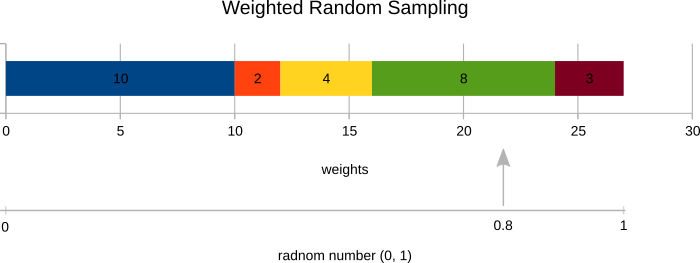Your implementation should return the same results:

>>> rng(0);
>>> distances = [10 2 4 8 3];
>>> idx = random_sample(distances)
>>> idx = random_sample(distances)

idx = 4

idx = 5

K-means++ favors initialization with cluster prototypes far from each other:

>>> rng(0);
>>> x = 1:100;
>>> means = k_meanspp(x, 3)

means =    82    46     3

Inspect the K-means++ initialization for the data generated by gen_kmeanspp_data.m and compare it to a uniform random initialization of vanilla K-means. Save the figures of initializations to random_initialization.png and kmeanspp_initialization.png. Use your random_sample function to generate ALL kmeans++ output indexes.

### 4. K-means Application - Color Quantization

Apply K-means algorithm to the color quantization problem. The aim is to reduce the number of distinct colors in an image such that the resulting image is visually close to the source image. The algorithm is as follows:

1. Convert the image from RGB color space to L*a*b* color space. Differences between colors measured as euclidean distances in the three-dimensional L*a*b* space correspond better to human perception of color differences.
2. Randomly select 1000 pixels from the input image.
3. Perform K-means clustering of the selected pixels in the three-dimensional L*a*b* space (using k_means function with max_iter=Inf, without k_meanspp initialization).
4. Assign all image pixels to the found means.
5. Convert the resulting image from the L*a*b* space back to the RGB space.

You are supposed to implement a function im_q = quantize_colors(im, k) which performs the described color quantization. Run this function on geeks.png image and save the resulting quantized version as geeks_quantized.png. The right image below is a sample result (for k=8):

Important (for automatic evaluation): To generate indices of the 1000 random pixels, use this code: inds = randsample(size(im,1) * size(im,2), 1000);. For python: inds = np.random.randint(0, (h_image * w_image)-1, 1000).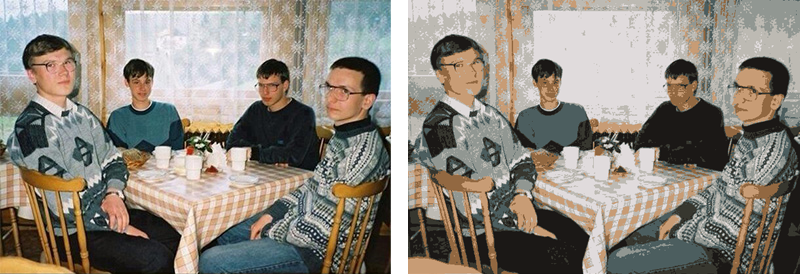## Assignment Questions

1. What is the theoretical shape of K-means clusters? What cluster shapes can be separated by the K-means algorithm the easiest?
• a) hypercube
• b) hyperellipsoid
• c) hypersphere
• d) hyperplane
2. Which of the following datasets would be correctly clustered using K-means with parameter k=2?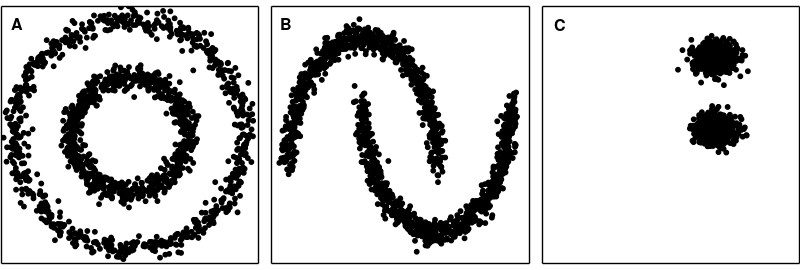3. Suppose that your are using slightly different version of the K-means random initialization. The initial means are just randomly generated vectors. This works reasonably well, but you can end up with an empty cluster while iterating. Choose two best options how to deal with an empty cluster:
• a) split the smallest cluster into two
• b) split the biggest cluster into two
• c) reduce the number of clusters
• d) randomly initialize the new mean
• e) assign mean of the whole dataset as the new mean
4. The sum of squared distances of the points to their assigned cluster means:
• a) Always decreases with the number of iterations
• b) Always increases with the number of iterations
• c) Can be non-monotonic in certain situations